Data and processes in computing

Start this free course now. Just create an account and sign in. Enrol and complete the course for a free statement of participation or digital badge if available.

# 2.5 Sequences

You have already met sequences briefly, and have seen that a sequence contains items given in a particular order, and that repetitions are of significance.

One might have a sequence containing integers, such as [22, −31, 44, 0, 2, 0, 11] or a sequence containing characters, such as [‘W’,‘o’,‘r’,‘d’]. However, we will aim to avoid mixing the forms of data in a sequence. A sequence of characters may also be referred to as a string, and that “Word” is another notation for the sequence [‘W’,‘o’,‘r’,‘d’].

The items in a sequence are enumerated from the left. Thus in the sequence [‘W’,‘o’,‘r’,‘d’], the first item is ‘W’, the second item is ‘o’, and so on. The items in a sequence may also be referred to as the elements (or sometimes members) of the sequence. The number of items in a sequence is called its length. So, for example, the length of the sequence [‘W’,‘o’,‘r’,‘d’] is 4.

We do count repeated items when calculating the length of a sequence. So, for example, the sequence of numbers [22, −31, 44, 0, 2, 0, 11] has length 7, while the sequence of characters “aardvark” has length 8.

Incidentally, we are only concerned in this course with finite sequences. If you study mathematics in other contexts, you may meet sequences that “go on forever”, such as the sequence of real numbers: 1,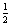,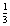,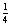,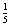, ... (where the general term is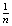where n is a positive integer). Any form of data stored on a computer as a sequence will contain a finite number of items, so a sequence describing a form of data stored on a computer will be finite. A finite sequence will have a length that is a positive integer.

## Activity 6

• (a) Let s be the sequence s = [44, 21, 77, 77, 41].

• (i) What is the third member of s?

• (ii) What is the fourth member of s?

• (iii) What is the length of s?

• (b) Let t be the sequence of characters t = “This sentence.”

• (i) What is the fifth member of t?

• (ii) What is the length of t?

• (iii) What is the fifteenth member of t?

• (c) Let A be the set of items appearing in the sequence s = [41, 21, 77, 77, 41]. What is the cardinality of A?

### Discussion

• (a)

• (i) The third member of s is 77.

• (ii) The fourth member of s is also 77.

• (iii) The sequence s contains 5 items, so the length of s is 5.

• (b)

• (i) The fifth member of t is the space character, which we write as ‘’.

• (ii) The length of t is 14. (You need to count the space character and the full stop as well as the letters.)

• (iii) There is no fifteenth character in t.

• (c) The set A takes no notice of the order in which the items appear in s, nor of repeated items. The set A is {21, 41, 77}, and this has cardinality 3.

The set A could equally well be written as {41, 21, 77}, for example.

We have introduced notations for the set of all integers, Int, and for the set of characters, Char. It is convenient also to have a notation for sequences. This will need to tell us two things: first, that we are referring to a set of sequences; and second, the set from which the members of the sequence come. Thus we write SeqOfInt for the set of all sequences of integers. So, for example, [22, −31, 44, 0, 2, 0, 11] is in SeqOfInt. Similarly, we write SeqOfChar for the set of all sequences of characters. The sequence [‘W’,‘o’,‘r’,‘d’] is in SeqOfChar (and not in SeqOfInt), because it is a sequence that contains characters, not integers.

There are a couple of points to note here. First, the number 22 is not in SeqOfInt, because 22 is not a sequence, it is an integer. However,  is in SeqOfInt. This is a sequence that happens to contain just one integer. Second, both SeqOfInt and SeqOfChar include an empty sequence, [].

More generally, we write SeqOfX for the set containing all sequences of items from the set X. Here X might be any set. For example, SeqOfBool contains all sequences whose members are from Bool, that is, whose members are Boolean values.

## Activity 7

• (a)

• (i) Is ‘a’ in the set SeqOfChar?

• (ii) Is “a” in the set SeqOfChar?

• (b) Give an example of a member of the set SeqOfBool.

### Discussion

• (a)

• (i) No. The single quotes here show that ‘a’ is a character, from the set Char. Just as there is a distinction between a single integer such as 22 and a sequence containing one integer such as , there is a corresponding distinction between a single character and a sequence containing one character.

• (ii) The double quotes here show we have a string; that is, a sequence of characters. So “a” is in SeqOfChar. The notation “a” means the same as [‘a’]: each is the sequence containing the single character ‘a’.

• (b) A suitable example is [true, true, false, true], but there are an infinite number of possible examples (including, if you chose to be contrary, the empty sequence [ ], containing no Boolean values!).